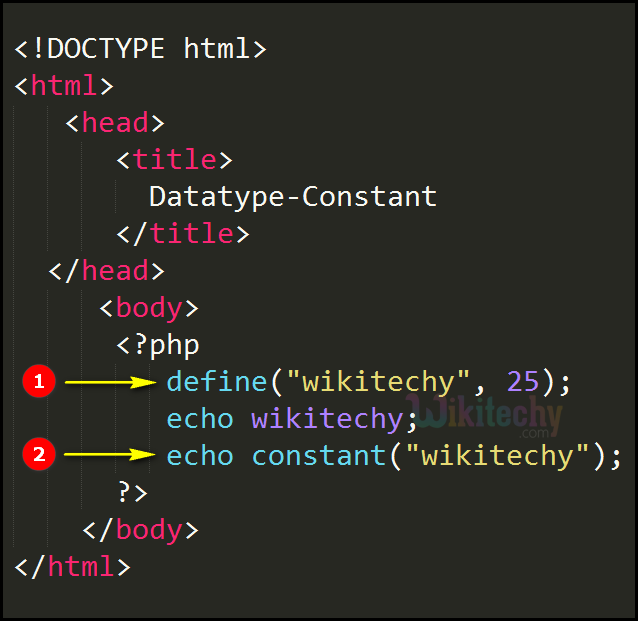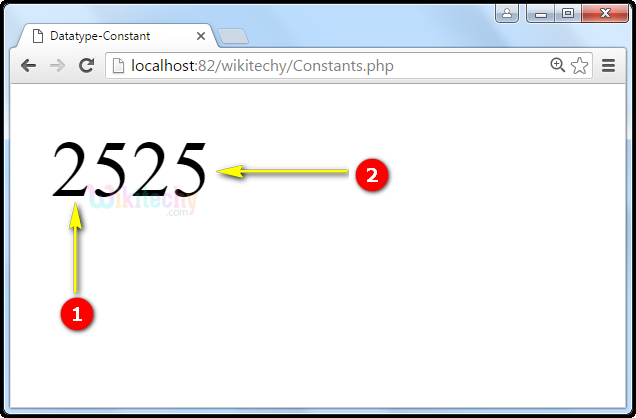# php tutorial - PHP Constants - php programming - learn php - php code - php script

• A constant is an identifier (identifier is the name that identifies labels, identity and so on). In general, the constant identifiers will always be in uppercase.
• In terms of the constant, its value cannot change during the execution of the script.
• 2 types of Constants are available:
1. Valid Constants
2. Invalid Constants

If a constant name starts with a letter or underscore, followed by any number of letters, numbers, or underscores then it is called as Valid Constants if not then it will be said to be Invalid Constant.

## Syntax

• name: Here the name variable specifies the name of the constant
• value: Here the value variable specifies the value of the constant.
• case-insensitive: This specifies whether the constant name should be case-insensitive or not .
• Constants are defined using define() function, which accepts two arguments:
• Name of the constant,
• And its value.
• Below is an example of defining a constant in a script shown below in the sample code.

## php examples Code Explanation :1. Here in this example of Php, define(“wikitechy”,25) it specifies the define() function that defines a constant with its name and value “25”.
2. echo constant(“wikitechy”) specifies the echo constant value is 25.

## php tutorials Sample Output :1. Output value “25” indicates the define() function which has a value(25) for the term wikitechy.
2. Output value “25” specifies the echo constant(“wikitechy”) whose value was already called as 25.

## Sample Code 2

• Create a constant with a case-sensitive name:

## Sample Code 3

• Create a constant with a case-insensitive name

## PHP Constant Arrays

• In PHP7, you can create an Array constant using the define() function.